Courses

# Systematic sampling (Part - 2), CSIR-NET Mathematical Sciences Mathematics Notes | EduRev

## Mathematics : Systematic sampling (Part - 2), CSIR-NET Mathematical Sciences Mathematics Notes | EduRev

The document Systematic sampling (Part - 2), CSIR-NET Mathematical Sciences Mathematics Notes | EduRev is a part of the Mathematics Course Mathematics for IIT JAM, CSIR NET, UGC NET.
All you need of Mathematics at this link: Mathematics

Comparison of systematic sampling, stratified sampling and SRS with population with linear trend: We assume that the values of units in the population increase according to linear trend. So the  values of successive units in the population increase in accordance with a linear model so that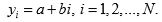Now we determine the variances of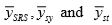under this linear  trend.

Under SRSWOR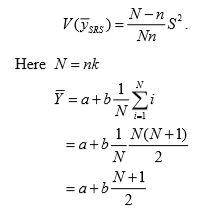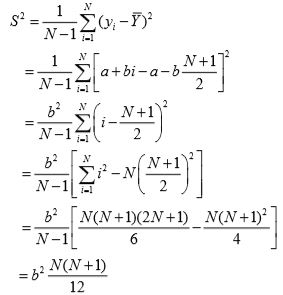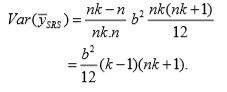Under systematic sampling Earlier yij denoted the value of study variable with the  jth unit in the ith systematic sample. Now yij represents the value of  [i + ( j− 1)k ]th unit of the population, so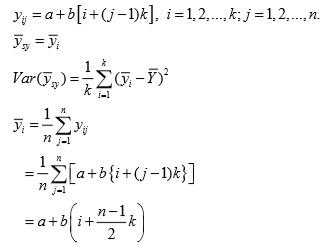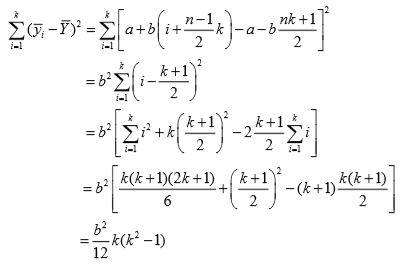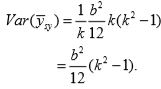Under stratified sampling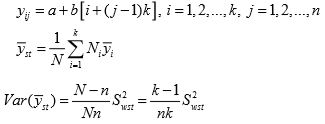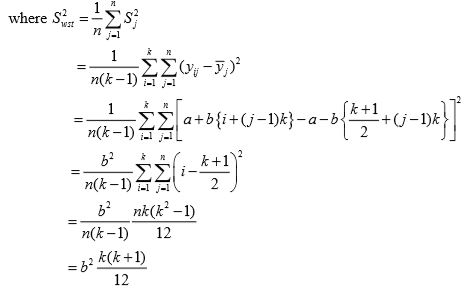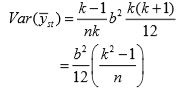If k is large, so that 1/k is negligible, then comparing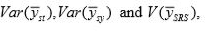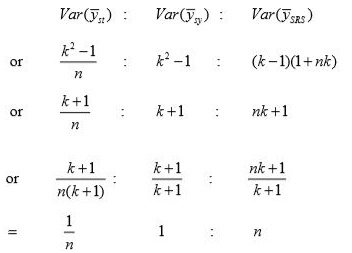Thus,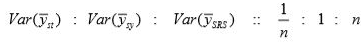So stratified  sampling is best for linearly trended population.  Next  best is systematic sampling.

Estimation of variance:

As such there is only one cluster, so variance in principle, cannot be estimated. Some approximations have been suggested.

1. Treat systematic sample as if it were a random sample. In this case, an estimate of variance is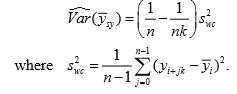This estimator under-estimates the true variance.

2. Use of successive differences of the values gives the estimate of variance as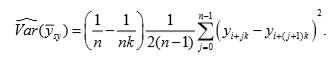This estimator is a biased estimator of true variance.

3. Use the balanced difference of  y1 ,y2 , ..., yn to get the estimate of variance as4. The interpenetrating subsamples can be utilized by dividing the sample into C groups each of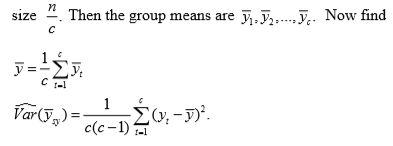Systematic sampling when  N ≠ nk.

When  N is not expressible as  nk then suppose  N can be expressed as N = nk + p; p< k.

Then consider the following sample mean as an estimator of population mean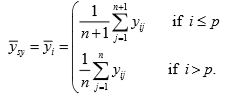In this case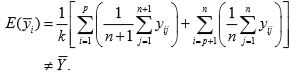So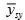is a biased estimator of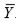.

An unbiased estimator ofis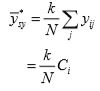where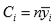is the total of values of the ith column.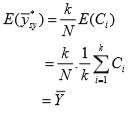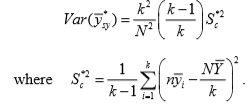Now we consider another procedure which is opted when N ≠ nk.

When population size  N is not expressible as the product of  n and  k , then let

N = nq + r.

Then take the sampling interval as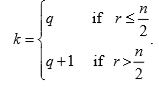Let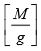denotes the largest integer contained in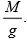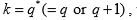number of units expected in sample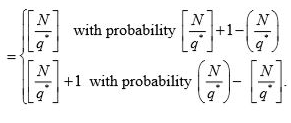If q = q*, then we get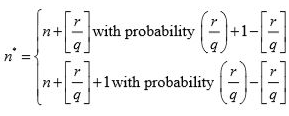Similarly if  = q* + 1, then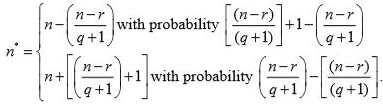Example: Let N = 17 and  n = 5. Then  q = 3 and r = 2 .  Since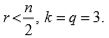Then sample sizes would be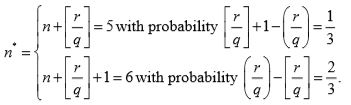This can be verified from the following example:

 Systematic sample number Systematic sample Probability 1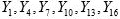1/3 2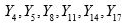1/3 3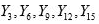1/3

We now prove the following theorem which shows how to obtain an unbiased estimator of the population mean when  N ≠ nk.

Theorem: In systematic sampling with sampling interval k from a population with size  N ≠ nk , an unbiased estimator of the population meanis given by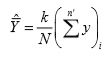where  i stands for the i th systematic sample, i = 1, 2, ..., k andn ' denotes the size of  ith systematic sample.

Proof. Each systematic sample has probability1/k.  Hence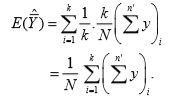Now, each unit occurs in only one of the k possible systematic samples. Hence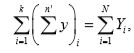which on substitution in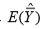proves the theorem.

When N ≠ nk , the systematic samples are not of the same size and the sample mean is not an unbiased estimator  of the population mean.  To overcome these disadvantages of systematic  sampling when N ≠ nk , circular systematic sampling is proposed. Circular  systematic sampling consists of selecting a random number from 1 to N and then selecting the unit corresponding to this random number.
Thereafter  every kth unit in a cyclical manner is selected till a sample of n units is obtained, k being the nearest integer to N/n

In other words, if  i is a number selected at random from 1 to N , then the circular systematic sample consists of units  with serial numbers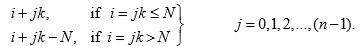This sampling scheme ensures equal probability of inclusion in the sample for every unit.

Example: Let N = 14 and n = 5. Then, k = nearest integer to 14/5 = 3 Let the first number selected at random from 1 to 14 be 7.  Then, the circular systematic sample consists of units with serial numbers 7,10,13, 16-14=2, 19-14=5.

This procedure is illustrated diagrammatically in following  figure.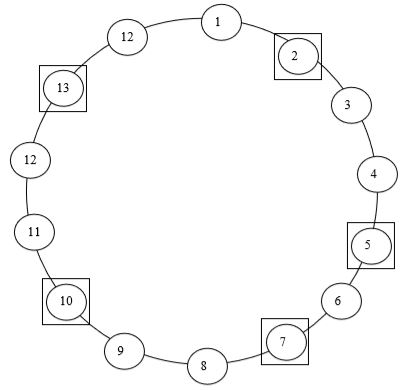Theorem: In circular systematic sampling, the sample mean is an unbiased estimator of the population mean.

Proof: If  i is the number selected at random, then the circular systematic sample mean is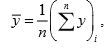where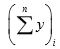denotes the total of  y values in the ith circular systematic sample, i = 1, 2, ...,N . We note here that in circular systematic sampling,  there are N circular systematic samples, each having probability 1/N of its selection.  Hence,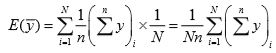Clearly, each unit of the population occurs in  n of the N possible circular systematic sample means.  Hence,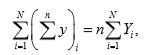which on substitution in E (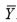) proves the theorem.

What to do when  N ≠ nk One of the following possible procedures may be adopted  when  N ≠ nk.

(i) Drop one unit at random if sample has (n + 1) units.
(ii) Eliminate some units so that N = nk .
(iii) Adopt circular systematic sampling scheme.
(iv) Round off the fractional interval k .

Offer running on EduRev: Apply code STAYHOME200 to get INR 200 off on our premium plan EduRev Infinity!

197 docs

,

,

,

,

,

,

,

,

,

,

,

,

,

,

,

,

,

,

,

,

,

,

,

,

;